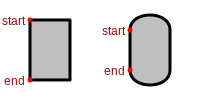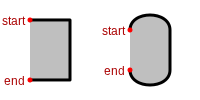# OE2DPath¶

```class OE2DPath
```

A sequence of lines and curves.

## Constructors¶

```OE2DPath()
```

Default constructor. The starting point of the path have to be defined using the `OE2DPath.AddStartPoint` method.

```OE2DPath(const OE2DPoint &start, bool closed=true)
```

Create a new path with a starting point.

start

The current point that will be starting point of the first line or curve.

closed

If true, than a line segment will be added from the starting point to the end point of the last line or curve segment of the path. See examples in Table: Examples of closed and open paths.

Note

The starting point of the path will be associated with the `OE2DPathPointType.Start` type when returned by the `OE2DPath.GetPoints` method.

Examples of closed and open paths

closed paths

open paths```OE2DPath(const OE2DPath &rhs)
```

Copy constructor.

```OE2DPath(const OE2DPath &rhs, double scale)
```

Copy constructor with scaling.

scale

The scaling factor is used to create a new image from the given one. The scaling factor has to be a positive (non-zero) number.

```OE2DPath(const OE2DPath &rhs, const OE2DPoint &offset)
```

Copy constructor with offset.

offset

The offset of new path relative to the copied one.

```OE2DPath(const OE2DPath &rhs, const OE2DPoint &center, double degrees)
```

Copy constructor with rotation.

center

The center of the rotation.

degree

The rotation in degrees.

## operator=¶

```OE2DPath &operator=(const OE2DPath &rhs)
```

Assignment operator.

```void AddCurveSegment(const OE2DPoint &c1, const OE2DPoint &c2,
const OE2DPoint &end)
```

Adds a cubic Bézier curve to the path from the end point of the last line or curve segment of the path to the position end using c1 and c2 control points.

c1, c2

The control points of the curve.

end

The end point of the curve segment.

Note

Points added to the path with the `OE2DPath.AddCurveSegment` method will be associated with the following types when returned by the `OE2DPath.GetPoints` method:

```void AddLineSegment(const OE2DPoint &end)
```

Adds a line to the path from the end point of the last line or curve segment of the path to the position end.

end

The end point of the line segment.

Note

Points added to the path with the `OE2DPath.AddLineSegment` method will be associated with the `OE2DPathPointType.LineEnd` type when returned by the `OE2DPath.GetPoints` method.

```void AddStartPoint(const OE2DPoint& start);
```

Adds the starting point of the path. If clears all previously added line or curve segments.

## GetEnd¶

```OE2DPoint  GetEnd() const;
```

Returns the end position of the path.

## GetPoints¶

```OESystem::OEIterBase<OE2DPathPoint> *GetPoints() const
```

Returns an iterator over all points of the path. Each point is associated with a type from the `OE2DPathPointType` namespace.

Example:

```foreach (OE2DPathPoint p in path.GetPoints())
{
OE2DPoint pos = new OE2DPoint(p.GetPoint());
Console.WriteLine(pos.GetX() + " " + pos.GetY() + " " + p.GetPointType());
}
```

## GetStart¶

```OE2DPoint  GetStart() const;
```

Returns the starting position of the path.

## IsClosed¶

```bool IsClosed() const
```

Returns whether a line segment will be added from the starting point to the end point of the last line or curve segment of the path. See examples in Table: Examples of closed and open paths.

## IsValid¶

```bool IsValid() const
```

Returns whether the `OE2DPath` object is valid. A path is considered valid if it has a starting point.

## SetClosed¶

```void SetClosed(bool closed)
```

Sets whether a line segment will be added from the starting point to the end point of the last line or curve segment of the path.